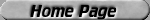Title: oneC_sum()

```A checksum is used to determine if errors occurred during the transmission of data. The checksum algorithm used by oneC_sum() (which is also the Internet standard) is described by RFC 1071. Essentially, the algorithm goes through data and adds all the bytes together (using one's complement addition). The high 16 bits of the resulting 32 bit value is then added to the low 16 bits (again, using one's complement addition). The checksum field is then set to the one's complement of this 16 bit sum. (Recall that the one's complement of 0xF0F0 is 0x0F0F.) Since AND'ing any 16 bit number and its 16 bit one's complement will equal 0xFFFF, the checksum of the packet (without the checksum field) AND'ed with the checksum field will equal 0xFFFF (provided the packet was not corrupted after the checksum field was calculated). For example, the checksum of a udp header (including the checksum field) will equal 0xFFFF if the packet was not corrupted in delivery. From RFC 1071: In outline, the Internet checksum algorithm is fairly simple: (1) Adjacent octets to be checksummed are paired to form 16-bit integers, and the 1's complement sum of these 16-bit integers is formed. (2) To generate a checksum, the checksum field itself is cleared, the 16-bit 1's complement sum is computed over the octets concerned, and the 1's complement of this sum is placed in the checksum field. (3) To check a checksum, the 1's complement sum is computed over the same set of octets, including the checksum field. If the result is all 1 bits (-0 in 1's complement arithmetic), the check succeeds. Below is a "C" code algorithm that describes the process above. This algorithm is also from RFC 1071. Note that count is the running count of all the bytes in the data and checksum is the return value. { /* Compute Internet Checksum for "count" bytes * beginning at location "addr". */ register long sum = 0; while( count > 1 ) { /* This is the inner loop */ sum += * (unsigned short) addr++; count -= 2; } /* Add left-over byte, if any */ if( count > 0 ) sum += * (unsigned char *) addr; /* Fold 32-bit sum to 16 bits */ while (sum>>16) sum = (sum & 0xffff) + (sum >> 16); checksum = ~sum; } ```